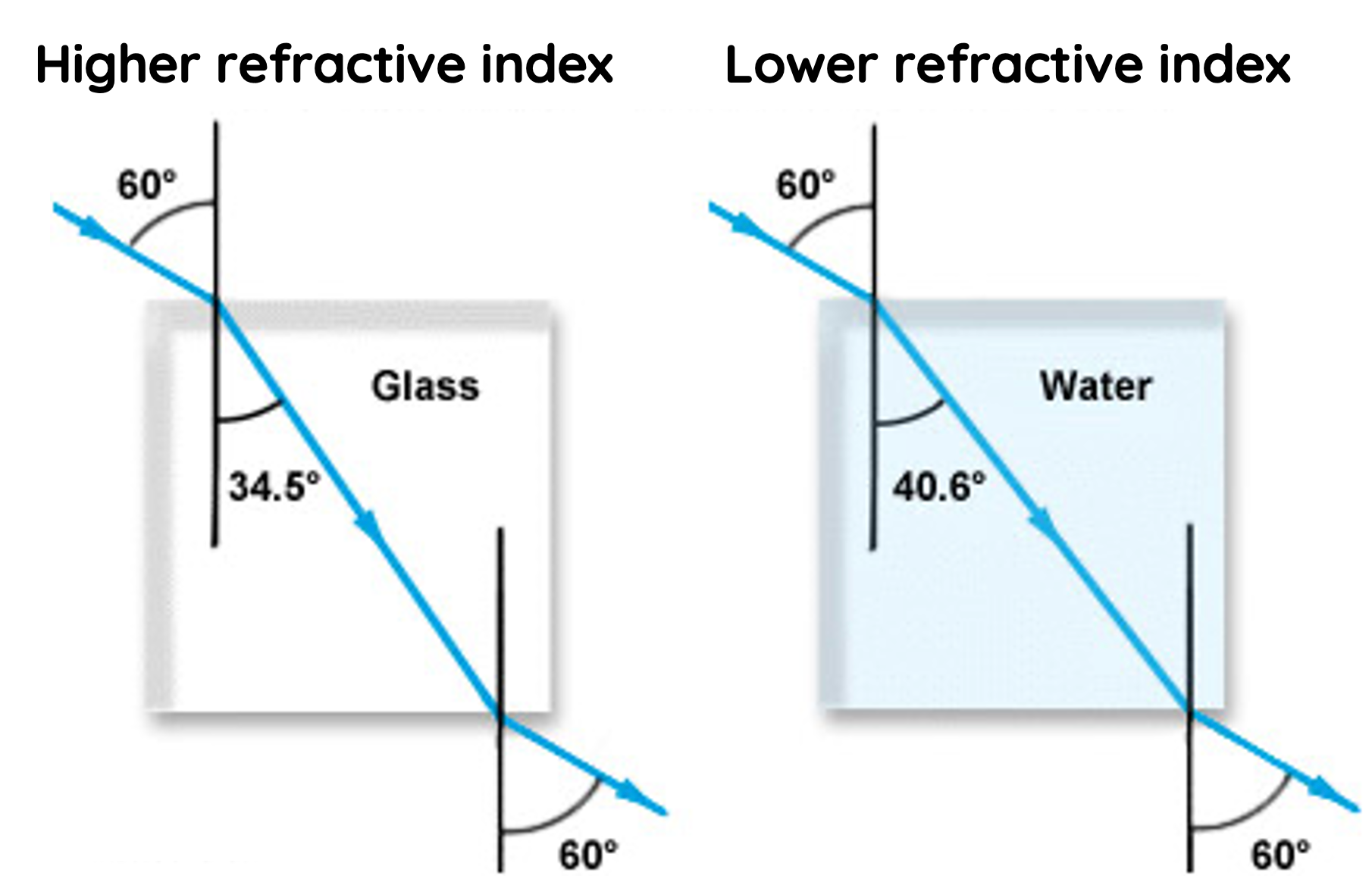# Refraction and Total Internal Reflection of light

This topic is part of the HSC Physics course under the section Ray Model of Light.

### HSC Physics Syllabus

• conduct investigations to examine qualitatively and quantitatively the refraction and total internal reflection of light (ACSPH075, ACSPH076)

• predict quantitatively, using Snell’s Law, the refraction and total internal reflection of light in a variety of situations

• solve problems or make quantitative predictions in a variety of situations by applying the following relationships to:

– n_x = c/{v_x} for the refractive index of medium x, v_x is the speed of light in the medium
– n_1 \sin \theta_1 = n_2 \sin \theta_2
– \sin \theta_c = n_2/{n_1}

### Refraction

Refraction is the bending of waves when they pass through different media, altering their speed and direction. Refraction of light can be understood using the ray model of light which helps visualise the direction of light rays.

Snell's Law

Snell's Law provides a mathematical way to predict the angle of refraction based on the medium's refractive index. It's expressed as:

$$n_1\sin{\theta_1} = n_2 \sin{\theta_2}$$

Where n_1 and n_2 are the refractive indices of the two media, and \theta_1 and \theta_2 are the angles of incidence and refraction, respectively.If the refractive index of the second medium is greater than the first medium, then the angle of refraction would be smaller than the angle of incidence. This means the wave would bend towards the normal as it travels through the medium.

Vice versa, if the refractive index of the second medium is smaller than the first medium, the wave would bend away from the normal as it travels through the medium.For a constant angle of incidence, the angle of refraction depends on the refractive index of the two medium. For example, light would have a smaller refractive angle in glass compared to water, because the refractive index of glass is greater than that of water.

Refraction: Effect on Speed, Frequency, and Wavelength

Refraction changes the speed of a wave as it passes from one medium into another, but it does so without altering the wave's frequency.

The speed of a wave is given by:

$$v = f \times \lambda$$

When a wave passes from one medium to another with a different refractive index, its velocity changes. The change in speed is due to a change in the wave's wavelength. The frequency of a light wave remains constant during refraction.

The velocity of light in a medium is given by:

$$v_x = \frac{c}{n_x}$$

where

– v_x is the velocity of light in the medium
– c is the velocity of light in a vacuum
– n_x is the refractive index of the medium

### Example 1 – Snell's Law

Light travels from air (n = 1.00) into an optical fibre with an index of refraction of 1.44.

(a) If the angle of incidence on the end of the fibre is 22º, what is the angle of refraction inside the fibre?

(b) What is the speed of light in the optic fibre?

Solution to part (a):

$$n_1\sin{\theta_1} = n_2 \sin{\theta_2}$$

$$(1.00)\sin{22º} = (1.44) \sin{\theta_2}$$

$$\theta_2 = 15º$$

Solution to part (b):

$$v_x = \frac{c}{n_x}$$

$$v_x = \frac{3 \times 10^8}{1.44} = 2.1 \times 10^8 \, \text{m/s}$$

### What is Critical Angle?

When light travels into a medium with a lower refractive index (less dense), it bends away from the normal. As the angle of incidence increases, the refracted light ray deviates further from the normal.The critical angle is the specific angle of incidence beyond which light cannot pass through a boundary between two media and is instead completely reflected within the more dense medium. This phenomenon is referred to as total internal reflection.

The critical angle can be calculated using Snell's Law, making the refracted angle equal to 90 degrees.

$$n_1\sin{\theta_1} = n_2 \sin{\theta_2}$$

$$n_1\sin{\theta_c} = n_2 \sin{90º}$$

$$\sin{\theta_c} = \frac{n_2}{n_1}$$

### Total Internal Reflection

Total internal reflection is the complete reflection of a wave within a medium when it reaches the boundary with a less dense medium at an angle greater than the critical angleIn total internal reflection, all the light is reflected back into the denser medium. None of it is refracted into the less dense medium.

### Example 2 – Total Internal Reflection

A ray of light makes a transition from a sample of benzene (n = 1.50) to water (n = 1.33).

(a) What is the critical angle?

(b) Explain why total internal reflection cannot occur when light travels from water to benzene.

Solution for part (a)

$$\sin{\theta_c} = \frac{n_2}{n_1}$$

$$\sin{\theta_c} = \frac{1.33}{1.50}$$

$$\theta_c = 62.5º$$

Solution for part (b)Total internal reflection can only occur when light travels from a medium of higher refractive index to a medium of lower refractive index.

### Applications of Total Internal Reflection

• Fibre Optic Communication: TIR is the fundamental principle behind fibre optic cables. Light signals are reflected internally within the core of the cable, allowing data to be transmitted over long distances with minimal loss. This is crucial in telecommunications and internet connectivity.
• Medical Endoscopes: Medical instruments like endoscopes utilise TIR to allow doctors to view inside the body without invasive surgery. Light is guided through the instrument to illuminate and project an internal image to an observer.
• Gemology: The brilliance and sparkle in cut gemstones, like diamonds, are enhanced through TIR. Proper cutting ensures that light entering the gemstone undergoes multiple internal reflections, maximizing its visible brilliance.